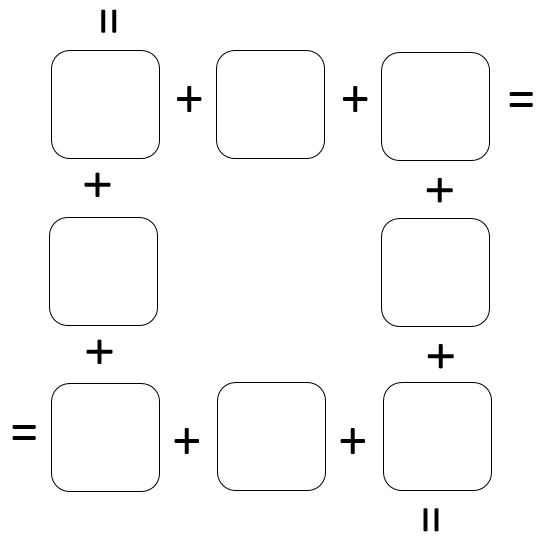Home > Grade 1 > Window Sum

# Window Sum

Directions: Using the digits 0-9, no more than once, complete the puzzle so that the sum of each side is equivalent.### Hint

The sum of the middle numbers on opposite sides are always the same

There are multiple answers, for example, you can arrange the numbers, 0, 1, 2, 3, 4, 5, 6, 7 in such a way that the sum of each side equals 9. Another solution includes using 0,5,7,3,2,6,4,8 and have sums of 12.

Source: Joshua Nelson and Renee Owen

## Open Number Line

Directions: Use the digits 1 to 9, at most one time each, place a digit …

1.0,7,3,2,6,5,1,4 and each side equals 9

•Reece Lane 7y.o.

If each sides equals 9 then it is 0,4,5,3,1,6,2,7
0+4+5=9
5+3+1=9
1+6+2=9
2+7+0=9

I don’t understand yours. 0,7,3,2,6,5,1,4. Can you explain please?
0+7+3=10
3+2+6=11
6+5+1=12
1+4+0=5

2.0,7,4,6,1,2,8,3 and each side equals 11.

3.Matthew McLaren

5,4,6,9,0,8,7,3

15

4.9 2 1
3 6
0 7 5